# KS1 Maths Quiz - Year 1 Measurements - Mass and Weight (Questions)

This quiz addresses the requirements of the National Curriculum KS1 Maths and numeracy for children aged 5 and 6 in year 1. Specifically this quiz is aimed at the section dealing with measuring and recording mass and weight.

Before they learn how to record the weight or mass of an item, children in Year 1 are encouraged to talk about 'heavier' and 'lighter' when comparing objects. Eventually, Year 1 children will begin to use standard units of measurement (kilograms and grams) to measure and record the weight of objects. They should also begin to be able to estimate the weight (or mass) of an item.

To see a larger image, click on the picture.
1. What weights do you see?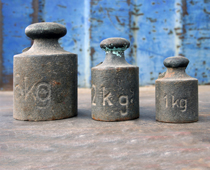[ ] 5kg, 4kg, 3kg [ ] 5kg, 2kg, 1kg [ ] 5kg, 12kg, 1kg [ ] 50kg, 20kg, 10kg
2. Which is the heaviest weight?[ ] 1kg [ ] 2kg [ ] 4kg [ ] 5kg
3. How much are peppers?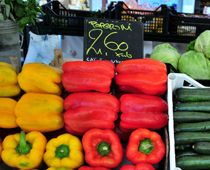[ ] £26.00 per kilogram [ ] £260 per kilogram [ ] 26p per kilogram [ ] £2.60 per kilogram
4. Which of the following would weigh about 1 kilogram?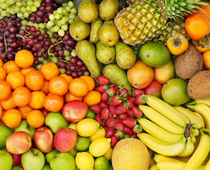[ ] 4 bananas [ ] 1 strawberry [ ] 4 grapes [ ] 1 apple
5. How much sugar might the girl use for a cake?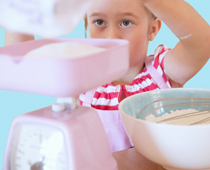[ ] 500g [ ] 500kg [ ] 5kg [ ] 5g
6. What could be on this scale?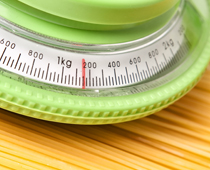[ ] A marble [ ] A feather [ ] An elephant [ ] 6 apples
7. How many kilograms does this scale weigh up to?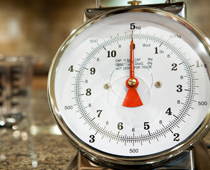[ ] 10kg [ ] 5kg [ ] 1kg [ ] 50kg
8. How much is shown on the scale?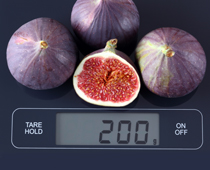[ ] 2kg [ ] 200g [ ] 200kg [ ] 20g
9. Do these pears weigh more than a kilogram?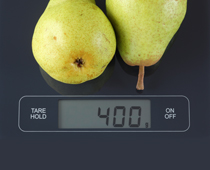[ ] Yes, they weigh 400kg [ ] No, 400g is less than half a kilogram [ ] Yes, they weigh 4kg [ ] Yes, they weigh 40kg
10. What could be on this scale?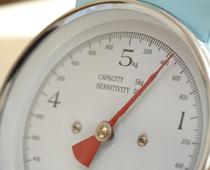[ ] A large bar of chocolate [ ] A cat [ ] A car [ ] A babyKS1 Maths Quiz - Year 1 Measurements - Mass and Weight (Answers)
1. What weights do you see?[ ] 5kg, 4kg, 3kg [x] 5kg, 2kg, 1kg [ ] 5kg, 12kg, 1kg [ ] 50kg, 20kg, 10kg
The largest weight is 5kg, the middle weight is 2kg and the smallest weight is 1kg
2. Which is the heaviest weight?[ ] 1kg [ ] 2kg [ ] 4kg [x] 5kg
The 5 kg is heavier than the others, and is larger too
3. How much are peppers?[ ] £26.00 per kilogram [ ] £260 per kilogram [ ] 26p per kilogram [x] £2.60 per kilogram
Fruit and vegetables sold by weight are priced per kilogram
4. Which of the following would weigh about 1 kilogram?[x] 4 bananas [ ] 1 strawberry [ ] 4 grapes [ ] 1 apple
4 bananas would be closer to 1 kg than all the others
5. How much sugar might the girl use for a cake?[x] 500g [ ] 500kg [ ] 5kg [ ] 5g
500g is also half a kilogram
6. What could be on this scale?[ ] A marble [ ] A feather [ ] An elephant [x] 6 apples
6 apples could weigh just over 1 kg, the others would not
7. How many kilograms does this scale weigh up to?[ ] 10kg [x] 5kg [ ] 1kg [ ] 50kg
The maximum weight on the scale is 5kg
8. How much is shown on the scale?[ ] 2kg [x] 200g [ ] 200kg [ ] 20g
200g is less than half a kilogram, which is 500g
9. Do these pears weigh more than a kilogram?[ ] Yes, they weigh 400kg [x] No, 400g is less than half a kilogram [ ] Yes, they weigh 4kg [ ] Yes, they weigh 40kg
They actually weigh less than half a kilogram!
10. What could be on this scale?[x] A large bar of chocolate [ ] A cat [ ] A car [ ] A baby
A large bar of chocolate would weigh just under half a kilogram, the others would be more - especially a car!